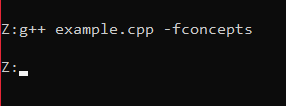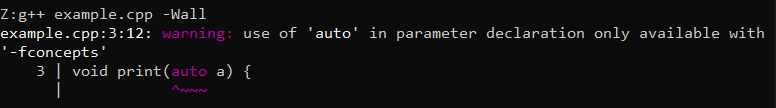# How to speed up g++ during compile time

• Last Updated : 18 Jan, 2021

Fast compilation on g++ build systems is basically used in compiling and executing C++ programs in the terminal. There are many options to speed up the compilation or even slow it down. Some of them are as follows:

### g++ {filename.cpp} -fconcepts:

• Compiling a program with fconcepts will not give any error as fconcepts ignores the warning.
• If the code is executed normally, it will give an error.
• In this way, fconcepts speed up the debugging.

Syntax:

g++ <filename.cpp> -fconcepts

Below is the C++ program to illustrate the use of -fconcepts:

## C++

 `// C++ program to illustrate the use ` `// of -fconcepts ` `#include ` `using` `namespace` `std; ` ` `  `// Function to print the integer a ` `void` `print(``auto` `a) ` `{ ` `    ``cout << a << endl; ` `} ` ` `  `// Driver Code ` `int` `main() ` `{ ` `    ``int` `a = 5; ` ` `  `    ``// Function Call ` `    ``print(a); ` ` `  `    ``return` `0; ` `}`

Output:Generally, functions do not allow an auto variable as function parameters. But using -fconcepts this error can be ignored.

### g++ {filename.cpp} -Wall:

• Compiling the same code using -Wall will give warnings as it will not ignore it.
• It also prompts as to where the code is wrong.

Syntax:

g++ <filename.cpp> -Wall

Below is the C++ program to illustrate the use of -Wall:

## C++

 `// C++ code to illustrate the use ` `// of -Wall ` `#include ` `using` `namespace` `std; ` ` `  `// Function to print a ` `void` `print(``auto` `a) ` `{ ` `    ``cout << a << endl; ` `} ` ` `  `// Driver Code ` `int` `main() ` `{ ` `    ``int` `a = 5; ` ` `  `    ``// Function call ` `    ``print(a); ` ` `  `    ``return` `0; ` `}`

Output:My Personal Notes arrow_drop_up
Recommended Articles
Page :